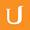## Complex Fractions Find the LCD 2 - Visualizing Algebra

• 0:00 - 0:06
The LCD would be x squared times x plus 2. Remember we want the highest power of
• 0:06 - 0:10
any factor that appears in a denominator. So we know x is already a factor of x
• 0:10 - 0:17
squared, so we should use x squared. And X plus two for our LCD. Another way to
• 0:17 - 0:22
think about this, is to use our factor tree method to find the LCD of X squared
• 0:22 - 0:27
and X plus two. We use X squared here, since we know X is already a factor of X
• 0:27 - 0:32
squared. Now don't make the mistake of putting X as the common factor here. X
• 0:32 - 0:38
plus two is one factor. Wgereas X squared has the factors of X and X. The only
• 0:38 - 0:44
common factor for X squared and the quantity X plus two is one. This also makes
• 0:44 - 0:50
sense because this X is a term inside this factor. So our LCD is X squared times
• 0:50 - 0:54
one times the quantity X plus two.
Title:
Complex Fractions Find the LCD 2 - Visualizing Algebra
Video Language:
English
Team:Udacity
Project:
MA006 - Visualizing Algebra
Duration:
0:54Udacity Robot edited English subtitles for Complex Fractions Find the LCD 2 - Visualizing AlgebraCogi-Admin added a translation

# English subtitles

## Revisions Compare revisions

• API
Udacity Robot